# Inverse Matrix Calculator

Select the number of equations in the matrix and enter the matrix values. Hit the Calculate button to calculate the inverse of a matrix using the calculator below.## Matrix Inverse Calculator

Inverse matrix calculator is an online tool that finds the inverse of a matrix for given values of a matrix. It can calculate the inverse of 2x2, 3x3, 4x4, and 5x5 matrices.

Let’s find out how to calculate inverse of matrix without using matrix inverse calculator.

## How to find the inverse of a matrix (3x3)?

System of equations in matrix can be solved using Crammer’s rule. To add, subtract or multiply matrices, matrix calculator could be a handy tool.

Inverse of matrix can be calculated using above calculator, but, here we will take the inverse manually.

Example:

Find the inverse of the following matrix A.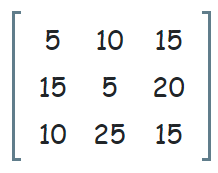Solution:

Step 1: Augment the matrix with identity matrix.Step 2: Make zeros in column 1 except the entry at row 1, column 1 (pivot entry).

Divide row 1 by 5 (R1/5)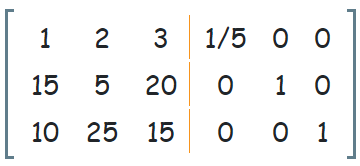R2 => R2 - 15R1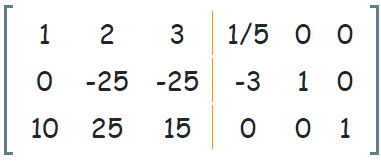R3 => R3 - 10R1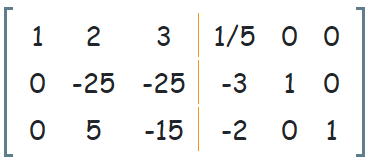Step 3: Make zeros in column 2 except the entry at row 2, column 2.

Divide row 2 by -25 (R2/-25)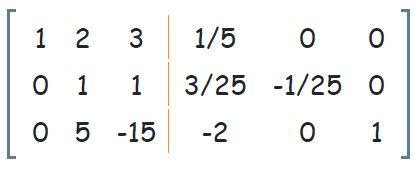R1 => R1 - 2R2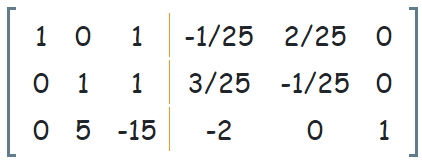R3 => R3 - 5R2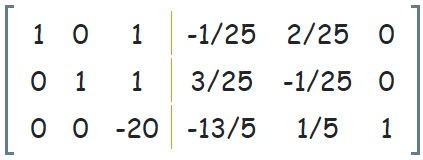Step 4: Make zeros in column 3 except the entry at row 3, column 3 (pivot entry).

Divide row 3 by -20 (R3/-20)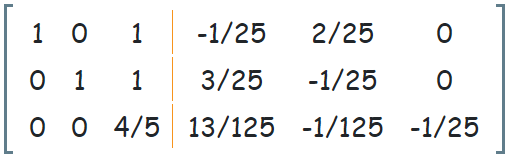R1 => R1 - 1R3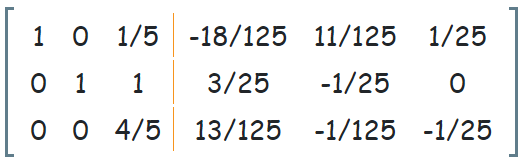R2 => R2 - 1R3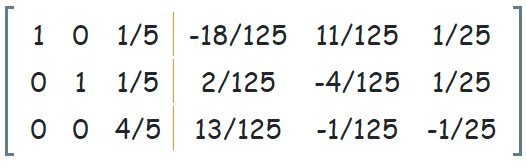The final A-1 can be written as,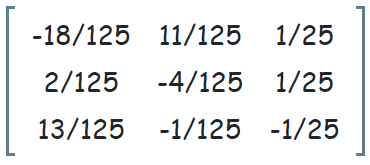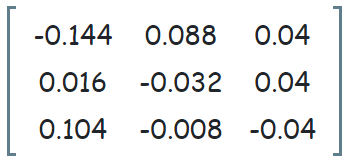1. Inverse of a Matrix by mathsisfun.com.
2. Mathwords: Inverse of a Matrix.